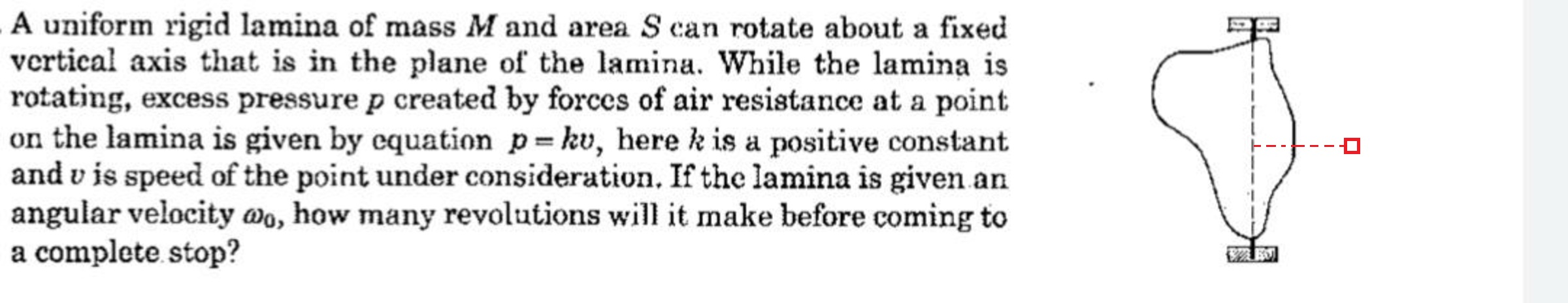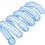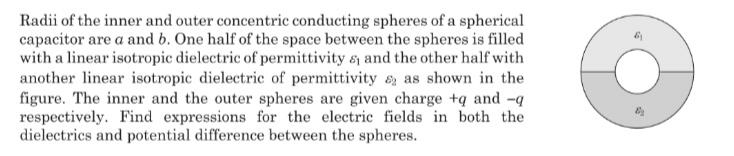# Rotation and Air ResistanceSince no specific shape is given, I will analyze a small square located a distance $$r$$ from the axis (shown in red in the diagram).

Moment of inertia:

$I = M r^2$

Pressure, force, and torque (let $\omega$ be the angular frequency):

$P = k v = k r \omega \\ F = P S = k S r \omega \\ \tau = F r = k S r^2 \omega$

Rotational Newton's 2nd Law:

$-\tau = I \dot{\omega} \\ -k S r^2 \omega = M r^2 \dot{\omega} \\ \dot{\omega} = - \frac{k S }{M} \omega$

This corresponds to exponential decay of the angular frequency:

$\omega = \omega_0 \, e^{-k S t/M}$

The angle swept out over infinite time is:

$\theta = \int_0^{\infty} \omega_0 \, e^{-k S t/M} \, dt = \frac{\omega_0 M}{k S}$

And the number of revolutions is therefore:

$N_{rev} = \frac{\theta}{2 \pi} = \frac{\omega_0 M}{2 \pi k S}$Note by Steven Chase
9 months ago

This discussion board is a place to discuss our Daily Challenges and the math and science related to those challenges. Explanations are more than just a solution — they should explain the steps and thinking strategies that you used to obtain the solution. Comments should further the discussion of math and science.

When posting on Brilliant:

• Use the emojis to react to an explanation, whether you're congratulating a job well done , or just really confused .
• Ask specific questions about the challenge or the steps in somebody's explanation. Well-posed questions can add a lot to the discussion, but posting "I don't understand!" doesn't help anyone.
• Try to contribute something new to the discussion, whether it is an extension, generalization or other idea related to the challenge.
• Stay on topic — we're all here to learn more about math and science, not to hear about your favorite get-rich-quick scheme or current world events.

MarkdownAppears as
*italics* or _italics_ italics
**bold** or __bold__ bold
- bulleted- list
• bulleted
• list
1. numbered2. list
1. numbered
2. list
Note: you must add a full line of space before and after lists for them to show up correctly
paragraph 1paragraph 2

paragraph 1

paragraph 2

[example link](https://brilliant.org)example link
> This is a quote
This is a quote
    # I indented these lines
# 4 spaces, and now they show
# up as a code block.

print "hello world"
# I indented these lines
# 4 spaces, and now they show
# up as a code block.

print "hello world"
MathAppears as
Remember to wrap math in $$ ... $$ or $ ... $ to ensure proper formatting.
2 \times 3 $2 \times 3$
2^{34} $2^{34}$
a_{i-1} $a_{i-1}$
\frac{2}{3} $\frac{2}{3}$
\sqrt{2} $\sqrt{2}$
\sum_{i=1}^3 $\sum_{i=1}^3$
\sin \theta $\sin \theta$
\boxed{123} $\boxed{123}$

## Comments

Sort by:

Top Newest

@Lil Doug Here it is

- 9 months ago

Log in to reply

@Steven Chase Thank you so much.

- 9 months ago

Log in to reply

@Steven Chase but one thing I want to ask how can we still call if no specific shape is given?

- 9 months ago

Log in to reply

I assume that means that the result holds for any shape. We could probably analyze a spinning disk and come up with the same result.

- 9 months ago

Log in to reply

@Steven Chase Very nice thanks .
By the way,Today I just read one fact on internet , and the fact is “If the people whose name starts with S helps the people whose name starts with N , then the people with S names get a lot of benefit in the Physics subject.

- 9 months ago

Log in to reply

@Steven Chase Take a look on this problem whenever you will be free, thanks in advance.If possible post a note. If it takes time to write latex , please use pen and page.
hope I am not disturbing you.

- 9 months ago

Log in to reply

×

Problem Loading...

Note Loading...

Set Loading...